Anzeige

# Physics project

3. Feb 2016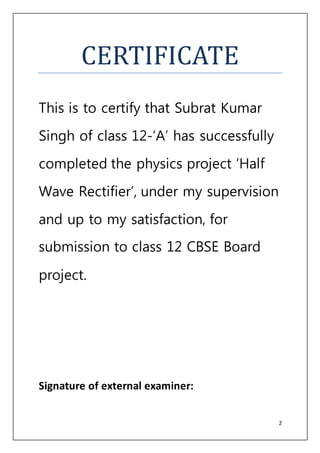Anzeige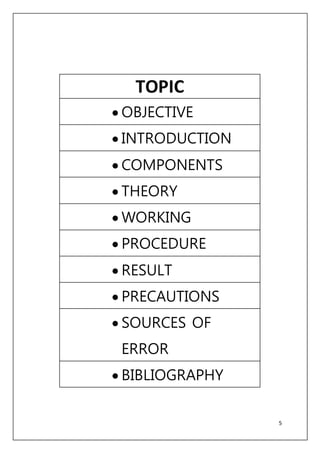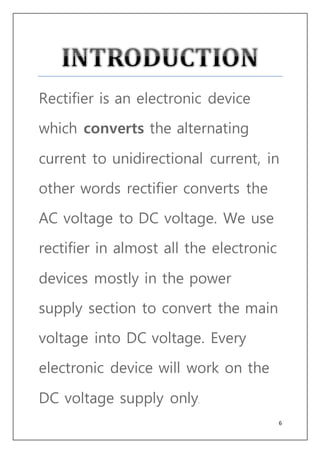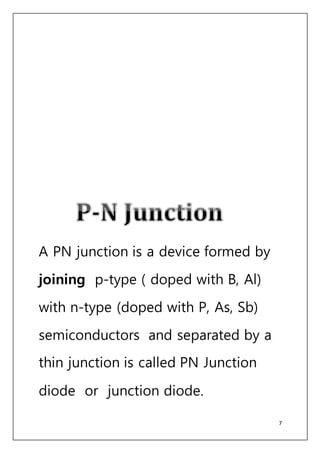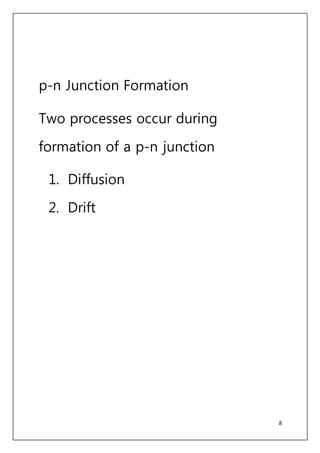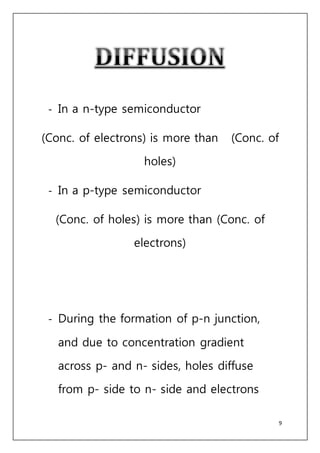Anzeige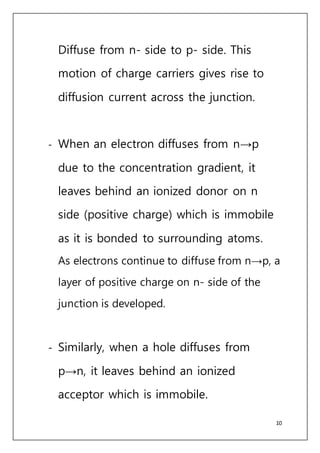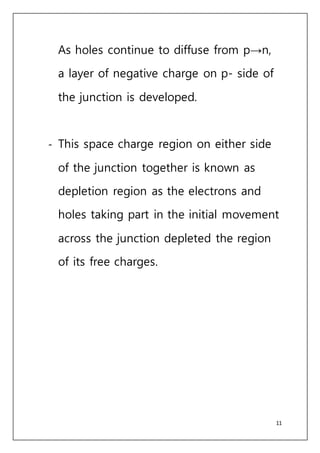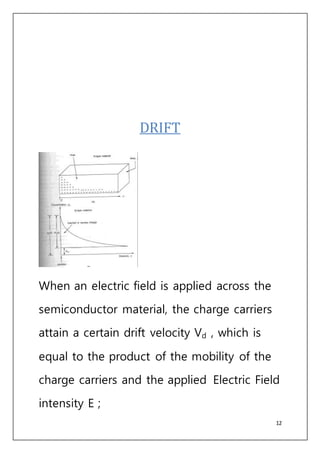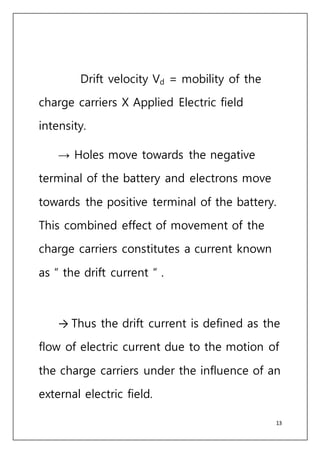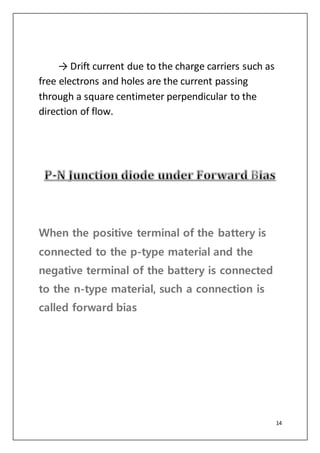Anzeige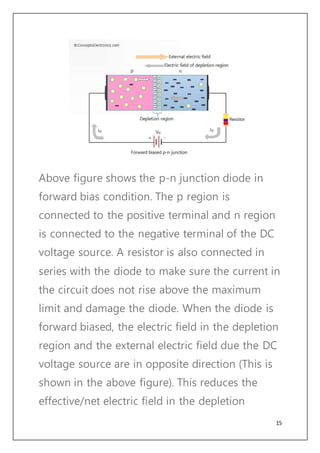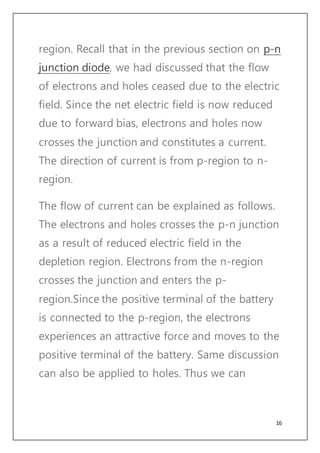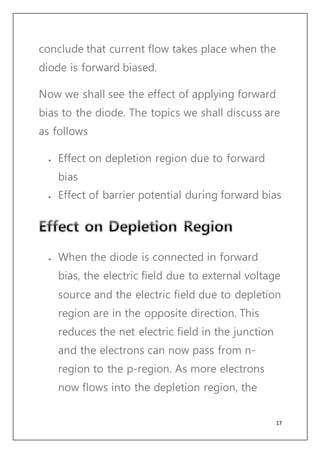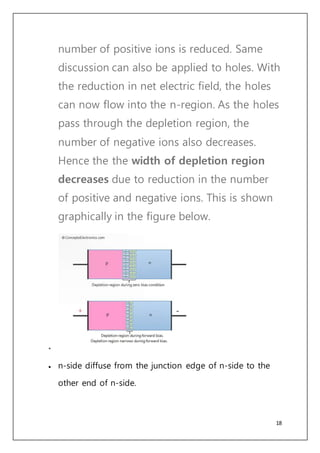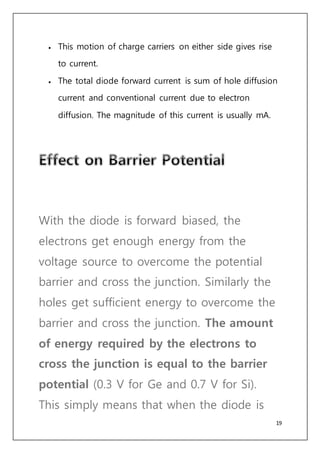Anzeige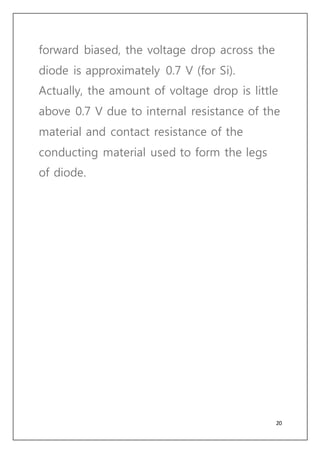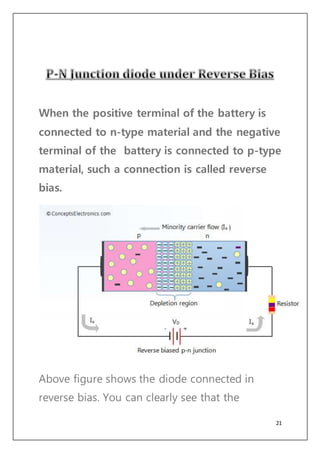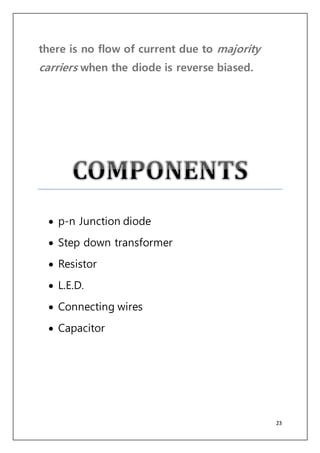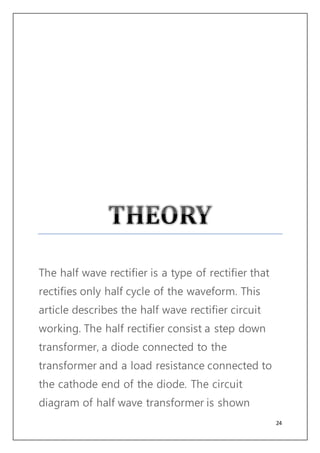Anzeige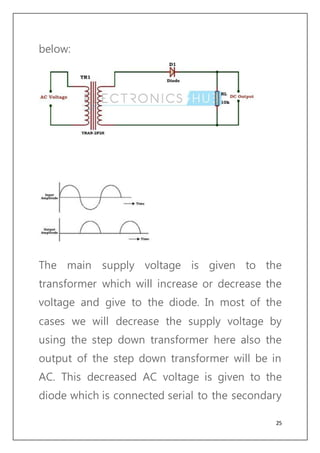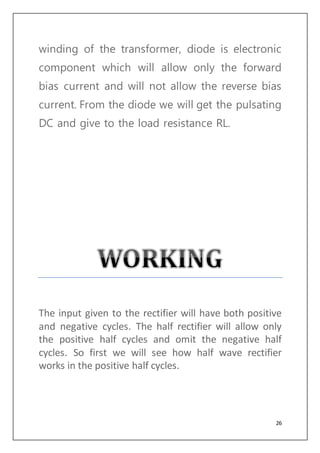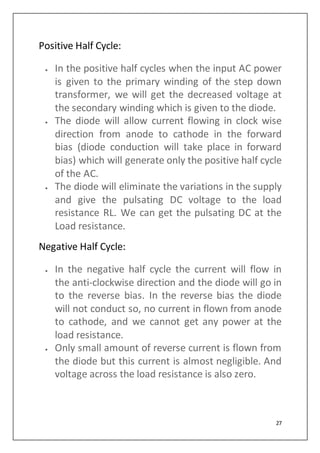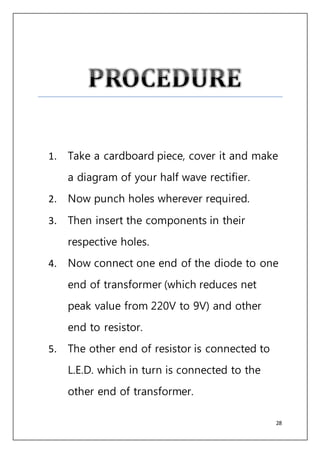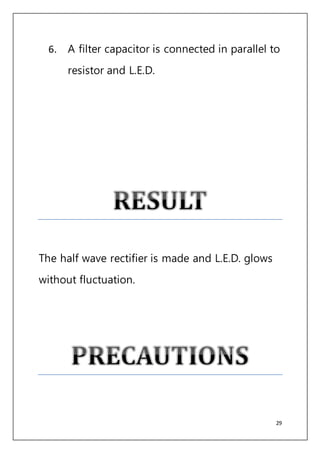Anzeige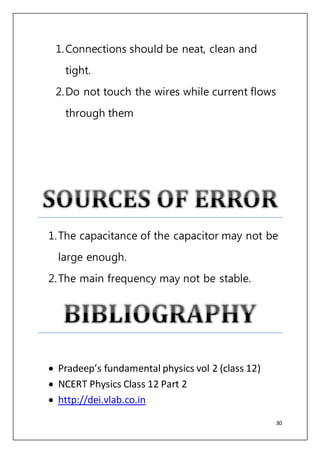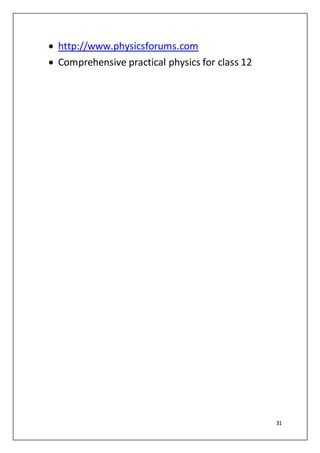Nächste SlideShare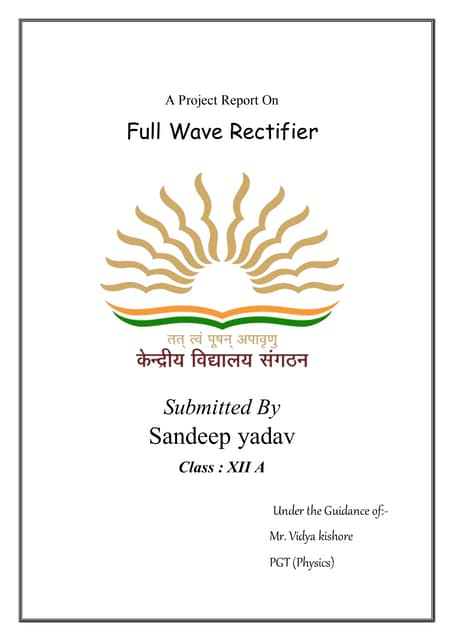Physics project
1 von 31
Anzeige

### Physics project

1. 1 SESSION 2015-2016 By-Subrat Kumar Singh School- Father Agnel School Roll no -32
2. 2 CERTIFICATE This is to certify that Subrat Kumar Singh of class 12-‘A’ has successfully completed the physics project ‘Half Wave Rectifier’, under my supervision and up to my satisfaction, for submission to class 12 CBSE Board project. Signature of external examiner:
3. 3 Signature (subject teacher): It gives me a great pleasure to express my gratitude and deepest respect to my physics teacher Mrs. Menka Prabhakar for her guidance and excellent supervision. Her constant encouragement only drove me to work, to bring out this work
4. 4 successfully. I would also like to thank our lab assistant Mr. Lalit for guiding me through this project. Finally, I wish to pay a glowing tribute to my parents for their love, affection and support.
5. 5 TOPIC  OBJECTIVE  INTRODUCTION  COMPONENTS  THEORY  WORKING  PROCEDURE  RESULT  PRECAUTIONS  SOURCES OF ERROR  BIBLIOGRAPHY
6. 6 Rectifier is an electronic device which converts the alternating current to unidirectional current, in other words rectifier converts the AC voltage to DC voltage. We use rectifier in almost all the electronic devices mostly in the power supply section to convert the main voltage into DC voltage. Every electronic device will work on the DC voltage supply only.
7. 7 A PN junction is a device formed by joining p-type ( doped with B, Al) with n-type (doped with P, As, Sb) semiconductors and separated by a thin junction is called PN Junction diode or junction diode.
8. 8 p-n Junction Formation Two processes occur during formation of a p-n junction 1. Diffusion 2. Drift
9. 9 - In a n-type semiconductor (Conc. of electrons) is more than (Conc. of holes) - In a p-type semiconductor (Conc. of holes) is more than (Conc. of electrons) - During the formation of p-n junction, and due to concentration gradient across p- and n- sides, holes diffuse from p- side to n- side and electrons
10. 10 Diffuse from n- side to p- side. This motion of charge carriers gives rise to diffusion current across the junction. - When an electron diffuses from n→p due to the concentration gradient, it leaves behind an ionized donor on n side (positive charge) which is immobile as it is bonded to surrounding atoms. As electrons continue to diffuse from n→p, a layer of positive charge on n- side of the junction is developed. - Similarly, when a hole diffuses from p→n, it leaves behind an ionized acceptor which is immobile.
11. 11 As holes continue to diffuse from p→n, a layer of negative charge on p- side of the junction is developed. - This space charge region on either side of the junction together is known as depletion region as the electrons and holes taking part in the initial movement across the junction depleted the region of its free charges.
12. 12 DRIFT When an electric field is applied across the semiconductor material, the charge carriers attain a certain drift velocity Vd , which is equal to the product of the mobility of the charge carriers and the applied Electric Field intensity E ;
13. 13 Drift velocity Vd = mobility of the charge carriers X Applied Electric field intensity. → Holes move towards the negative terminal of the battery and electrons move towards the positive terminal of the battery. This combined effect of movement of the charge carriers constitutes a current known as “ the drift current “ . → Thus the drift current is defined as the flow of electric current due to the motion of the charge carriers under the influence of an external electric field.
14. 14 → Drift current due to the charge carriers such as free electrons and holes are the current passing through a square centimeter perpendicular to the direction of flow. When the positive terminal of the battery is connected to the p-type material and the negative terminal of the battery is connected to the n-type material, such a connection is called forward bias
15. 15 Above figure shows the p-n junction diode in forward bias condition. The p region is connected to the positive terminal and n region is connected to the negative terminal of the DC voltage source. A resistor is also connected in series with the diode to make sure the current in the circuit does not rise above the maximum limit and damage the diode. When the diode is forward biased, the electric field in the depletion region and the external electric field due the DC voltage source are in opposite direction (This is shown in the above figure). This reduces the effective/net electric field in the depletion
16. 16 region. Recall that in the previous section on p-n junction diode, we had discussed that the flow of electrons and holes ceased due to the electric field. Since the net electric field is now reduced due to forward bias, electrons and holes now crosses the junction and constitutes a current. The direction of current is from p-region to n- region. The flow of current can be explained as follows. The electrons and holes crosses the p-n junction as a result of reduced electric field in the depletion region. Electrons from the n-region crosses the junction and enters the p- region.Since the positive terminal of the battery is connected to the p-region, the electrons experiences an attractive force and moves to the positive terminal of the battery. Same discussion can also be applied to holes. Thus we can
17. 17 conclude that current flow takes place when the diode is forward biased. Now we shall see the effect of applying forward bias to the diode. The topics we shall discuss are as follows  Effect on depletion region due to forward bias  Effect of barrier potential during forward bias  When the diode is connected in forward bias, the electric field due to external voltage source and the electric field due to depletion region are in the opposite direction. This reduces the net electric field in the junction and the electrons can now pass from n- region to the p-region. As more electrons now flows into the depletion region, the
18. 18 number of positive ions is reduced. Same discussion can also be applied to holes. With the reduction in net electric field, the holes can now flow into the n-region. As the holes pass through the depletion region, the number of negative ions also decreases. Hence the the width of depletion region decreases due to reduction in the number of positive and negative ions. This is shown graphically in the figure below.   n-side diffuse from the junction edge of n-side to the other end of n-side.
19. 19  This motion of charge carriers on either side gives rise to current.  The total diode forward current is sum of hole diffusion current and conventional current due to electron diffusion. The magnitude of this current is usually mA. With the diode is forward biased, the electrons get enough energy from the voltage source to overcome the potential barrier and cross the junction. Similarly the holes get sufficient energy to overcome the barrier and cross the junction. The amount of energy required by the electrons to cross the junction is equal to the barrier potential (0.3 V for Ge and 0.7 V for Si). This simply means that when the diode is
20. 20 forward biased, the voltage drop across the diode is approximately 0.7 V (for Si). Actually, the amount of voltage drop is little above 0.7 V due to internal resistance of the material and contact resistance of the conducting material used to form the legs of diode.
21. 21 When the positive terminal of the battery is connected to n-type material and the negative terminal of the battery is connected to p-type material, such a connection is called reverse bias. Above figure shows the diode connected in reverse bias. You can clearly see that the
22. 22 negative terminal of the battery is connected to p-type material and the positive terminal of the battery is connected to n-type material. A resistor is also connected in series with the diode, although resistor is not required when the diode is reverse biased. When the diode is reverse biased, the electric field due to the battery and the electric field of the depletion region are in the same direction. This makes the electric field even stronger than that before reverse bias was applied. The electrons from the n-type material (majority carriers) now faces a stronger electric field and it becomes even more difficult for them to move towards the p-type material. Same discussion also applies to holes. The holes from the p-type material (majority carriers) now faces a stronger electric field and it becomes even more difficult to move from p- type to n-type material.Hence we conclude that
23. 23 there is no flow of current due to majority carriers when the diode is reverse biased.  p-n Junction diode  Step down transformer  Resistor  L.E.D.  Connecting wires  Capacitor
24. 24 The half wave rectifier is a type of rectifier that rectifies only half cycle of the waveform. This article describes the half wave rectifier circuit working. The half rectifier consist a step down transformer, a diode connected to the transformer and a load resistance connected to the cathode end of the diode. The circuit diagram of half wave transformer is shown
25. 25 below: The main supply voltage is given to the transformer which will increase or decrease the voltage and give to the diode. In most of the cases we will decrease the supply voltage by using the step down transformer here also the output of the step down transformer will be in AC. This decreased AC voltage is given to the diode which is connected serial to the secondary
26. 26 winding of the transformer, diode is electronic component which will allow only the forward bias current and will not allow the reverse bias current. From the diode we will get the pulsating DC and give to the load resistance RL. The input given to the rectifier will have both positive and negative cycles. The half rectifier will allow only the positive half cycles and omit the negative half cycles. So first we will see how half wave rectifier works in the positive half cycles.
27. 27 Positive Half Cycle:  In the positive half cycles when the input AC power is given to the primary winding of the step down transformer, we will get the decreased voltage at the secondary winding which is given to the diode.  The diode will allow current flowing in clock wise direction from anode to cathode in the forward bias (diode conduction will take place in forward bias) which will generate only the positive half cycle of the AC.  The diode will eliminate the variations in the supply and give the pulsating DC voltage to the load resistance RL. We can get the pulsating DC at the Load resistance. Negative Half Cycle:  In the negative half cycle the current will flow in the anti-clockwise direction and the diode will go in to the reverse bias. In the reverse bias the diode will not conduct so, no current in flown from anode to cathode, and we cannot get any power at the load resistance.  Only small amount of reverse current is flown from the diode but this current is almost negligible. And voltage across the load resistance is also zero.
28. 28 1. Take a cardboard piece, cover it and make a diagram of your half wave rectifier. 2. Now punch holes wherever required. 3. Then insert the components in their respective holes. 4. Now connect one end of the diode to one end of transformer (which reduces net peak value from 220V to 9V) and other end to resistor. 5. The other end of resistor is connected to L.E.D. which in turn is connected to the other end of transformer.
29. 29 6. A filter capacitor is connected in parallel to resistor and L.E.D. The half wave rectifier is made and L.E.D. glows without fluctuation.
30. 30 1.Connections should be neat, clean and tight. 2.Do not touch the wires while current flows through them 1.The capacitance of the capacitor may not be large enough. 2.The main frequency may not be stable.  Pradeep’s fundamental physics vol 2 (class 12)  NCERT Physics Class 12 Part 2  http://dei.vlab.co.in
31. 31  http://www.physicsforums.com  Comprehensive practical physics for class 12
Anzeige